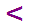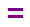Skill 6B
< > = Comparing Numbers: 30 to 100

Same skill as 6A, however, now we test student understanding of numbers 30 to 100.

Below each problem are three symbols used to compare numbers.  I want you to read the number sentence and use either the words -
is more than, is less than, or is equal to to make the number sentence TRUE.  Then point to the correct symbol below the number sentence.
Again, if your student cannot do exactly this, the skill must be practiced. It is not sufficient if your student just points to the correct symbol.

 785689899748